Start typing, then use the up and down arrows to select an option from the list.Jules Bruno
226views
Hey, guys, In this new video, we're gonna put to practice some of the principles we learned in creating a buffer. So, for example, when it says which of the following combinations can result in the formation of a buffer, so we should realize here is in every example, we have 0.1 moles off Hypo Cloris acid now hypochlorite acid, it says acid. So we know it's some type of acid. It has oxygen, so it's an oxy acid. Remember, if we do the math one oxygen minus one hydrogen gives us zero left over, so hypochlorite sass. It is a weak acid, so every single one of these is a weak acid. And because of that, you have to remember what two ways can we make a buffer if we have a weak acid? So remember the two ways is if we have a weak acid and its conjugate base, and the second ways if we have a weak acid and a strong base, But in this second case, the week species has to be higher in amount. So let's take a look at the what's mixing with here. We have a wage which is a strong base. Here we have HCL, which is a strong binary acid. Here in H three is a neutral amine. So it's a week base and anyway, which again is a strong base. So we're looking for the weak acid to be paired up with the conjugate base or the strong base so automatically these tour out because we can't pair up a weak acid with a strong acid or weak acid with a weak base has to be a conjugal base or strong base. Now, in the first one, we actually have more moles of the strong species, so this would not be a buffer. This would actually destroy the buffer. But for the last one, we have mawr of the week species than the strong species, so it would be the last one that makes a buffer. So again, remember the three principles that we learned in creating a buffer apply them to this type of question. Now, for practice one, I want you guys to figure out which one of these combinations will result in a buffer here. Remember, the word off means multiply. So if you multiply these two numbers together, you can help to find moles because remember, moles equals leaders times molar ity. So divide each of these by 1000 multiplied by the molar iti to have moles. You could also keep it in milliliters and multiply times the polarity. But then that would give you Millie moles. So I don't know if you guys want to deal with Millie moles. It's basically the same thing, but you don't have to do it that way, So divide them by 1000. The MLS to get leaders multiply times similarity to give you moles. Remember the three combinations that could result in the formation of a buffer. So check for each one of these. Look to see. Do you have a weak acid and conjugate base? Do you have a weak acid and strong base, or do you have a strong acid and weak base? Look for these combinations. If you don't see them, don't bother doing the math. So I would recommend looking at each compound first and seeing if they match up with any of these combinations. If they do look more deeply look to see doesn't help to create a buffer. If it doesn't match one of these three combinations, don't even bother looking at it. Don't do the math at all. And remember one key thing. I'll give you guys a hint. You have to find out to make sure that the you have the correct concentration of your strong bases. What did we say about strong basis? The concentration I give you is dependent on how maney ions you have, of which particular four ions doing that will help toe get the correct answer. Once you're done with this, come back and click on the explanation button and watch me explain how toe best approach this problem. Good luck, guys.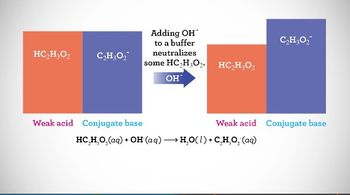6:19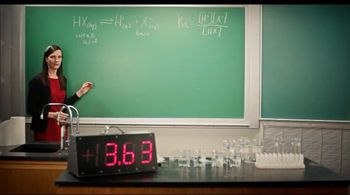5:12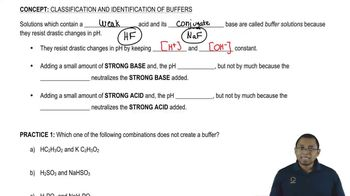03:14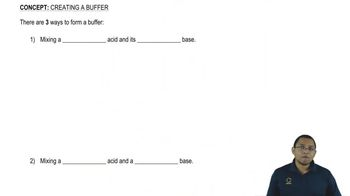04:34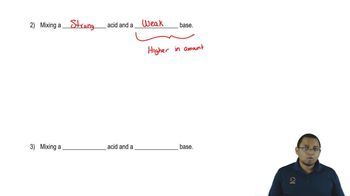01:44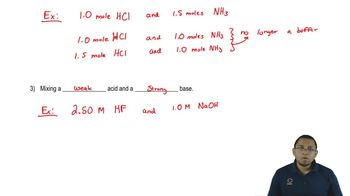01:33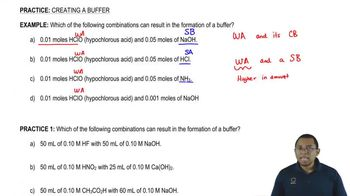03:53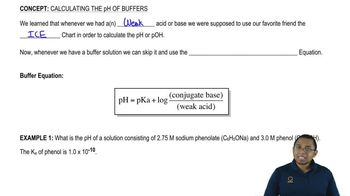01:19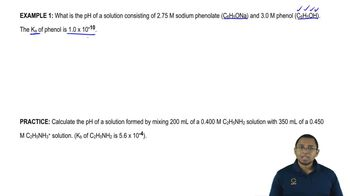02:33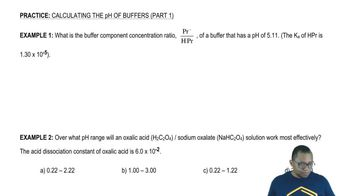03:22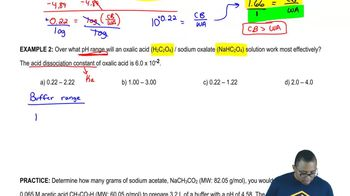02:24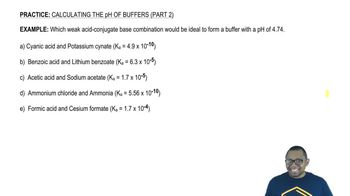02:33